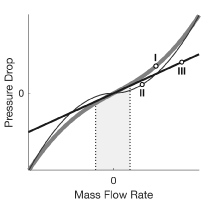# Flow Resistance (2P)

General resistance in a two-phase fluid branch

• Library:
• Simscape / Foundation Library / Two-Phase Fluid / Elements

•## Description

The Flow Resistance (2P) block models a general pressure drop in a two-phase fluid network branch. The pressure drop is proportional to the square of the mass flow rate and to the density of the two-phase fluid. The constant of proportionality is determined from a nominal operating condition specified in the block dialog box.

Use this block when the only data available for a component is its pressure drop as a function of its mass flow rate. Combine the block with others to create a custom component that more accurately captures the pressure drop that it induces—for example, a heat exchanger based on a chamber block.

### Mass Balance

The volume of fluid inside the flow resistance is assumed to be negligible. The mass flow rate in through one port must then exactly equal the mass flow rate out through the other port:

`${\stackrel{˙}{m}}_{\text{A}}+{\stackrel{˙}{m}}_{\text{B}}=0,$`

where ${\stackrel{˙}{m}}_{\text{A}}$ and ${\stackrel{˙}{m}}_{\text{B}}$ are defined as the mass flow rates into the component through ports A and B, respectively.

### Energy Balance

Energy can enter and leave the flow resistance through the two-phase fluid ports only. No heat exchange occurs between the wall and the environment. In addition, no work is done on or by the fluid. The energy flow rate in through one port must then exactly equal the energy flow rate out through the other port:

`${\varphi }_{\text{A}}+{\varphi }_{\text{B}}=0,$`

where ϕA and ϕB are the energy flow rates into the flow resistance through ports A and B.

### Momentum Balance

The relevant external forces on the fluid include those due to pressure at the ports and those due to viscous friction at the component walls. Gravity is ignored as are other body forces. Expressing the frictional forces in terms of a loss factor ξ yields the semi-empirical expression:

`$\Delta p=\xi \frac{\nu {\stackrel{˙}{m}}^{2}}{2{S}^{2}},$`

where:

• Δp is the pressure drop from port A to port B—that is, pA + pB.

• ξ is the loss factor.

• ν is the specific volume, the inverse of the mass density ρ—that is, 1/ρ.

• S is the flow area.

The pressure drop equation is implemented with two modifications. First, to allow for a change in sign upon reversal of flow direction, it is rewritten:

`$\Delta p=\xi \frac{\nu \stackrel{˙}{m}|\stackrel{˙}{m}|}{2{S}^{2}},$`

where the pressure drop is positive only if the mass flow rate is too. Second, to eliminate singularities due to flow reversal—singularities that can pose a challenge for numerical solvers during simulation—it is linearized in a small region of near-zero flow:

`$\Delta p=\xi \frac{\nu \stackrel{˙}{m}\sqrt{{\stackrel{˙}{m}}^{2}+\text{​}{\stackrel{˙}{m}}_{\text{Th}}^{2}}}{2{S}^{2}},$`

where ${\stackrel{˙}{m}}_{Th}$ is a threshold mass flow rate below which the pressure drop is linearized. The figure shows the modified pressure drop against the local mass flow rate (curve I):

• Above ${\stackrel{˙}{m}}_{Th}$, the pressure drop approximates that expressed in the original equation (curve II) and it varies with ${\stackrel{˙}{m}}^{2}$. This dependence is commensurate with that observed in turbulent flows.

• Below ${\stackrel{˙}{m}}_{Th}$, the pressure drop approximates a straight line with slope partly dependent on ${\stackrel{˙}{m}}_{Th}$ (curve III) and it varies with $\stackrel{˙}{m}$. This dependence is commensurate with that observed in laminar flows.For ease of modeling, the loss factor ξ is not required as a block parameter. Instead, it is automatically computed from the nominal condition specified in the block dialog box:

`$\frac{\xi }{2{S}^{2}}=\frac{\Delta {p}_{*}}{{\nu }_{*}{\stackrel{˙}{m}}_{*}^{2}},$`

where the asterisk (*) denotes a value at the nominal operating condition. Underlying all of these calculations is the assumption that the threshold mass flow rate ${\stackrel{˙}{m}}_{Th}$ is much smaller than the nominal value ${\stackrel{˙}{m}}_{*}$. Replacing the fraction ξ/(2S2) in the expression for the pressure drop yields:

`$\Delta p=\frac{\nu \Delta {p}_{*}}{{\nu }_{*}{\stackrel{˙}{m}}_{*}^{2}}\left(\stackrel{˙}{m}\sqrt{{\stackrel{˙}{m}}^{2}+{\stackrel{˙}{m}}_{\text{Th}}^{2}}\right).$`

or, equivalently:

`$\Delta p=C\nu \stackrel{˙}{m}\sqrt{{\stackrel{˙}{m}}^{2}+{\stackrel{˙}{m}}_{\text{Th}}^{2}},$`

where C is a constant of proportionality between the pressure drop across the flow resistance and the local mass flow rate. It is defined as:

`$C=\frac{\Delta {p}_{*}}{{\nu }_{*}{\stackrel{˙}{m}}_{*}^{2}}.$`

If the specific volume—and therefore the mass density—is assumed to be invariant, then its nominal and actual values must always be equal. This is the case whenever the nominal value is specified in the block dialog box as `0`—a special value used to signal to the block that the specific volume is a constant. The ratio of the two is then `1` and the product Cν reduces to:

`$C\nu =\frac{\Delta {p}_{*}}{{\stackrel{˙}{m}}_{*}^{2}}.$`

## Ports

### Conserving

expand all

Port through which the two-phase fluid enters or exits the flow resistance.

Port through which the two-phase fluid enters or exits the flow resistance.

## Parameters

expand all

Pressure drop from inlet to outlet at a known operating condition. The block uses the nominal parameters to calculate the constant of proportionality between the pressure drop and the mass flow rate.

Mass flow rate through the component at a known operating condition. The block uses the nominal parameters to calculate the constant of proportionality between the pressure drop and the mass flow rate.

Specific volume inside the flow resistance at a known operating condition. The block uses the nominal parameters to calculate the constant of proportionality between the pressure drop and the mass flow rate. Set this parameter to zero to ignore the dependence of the pressure drop on the specific volume.

Flow area at the ports of the flow resistance. The ports are assumed to be identical in size.

Ratio of the threshold mass flow rate to the nominal mass flow rate. The block uses this parameter to calculate the threshold mass flow rate—and ultimately to set the limits of linearization for the pressure drop.

### Variables

Mass flow rate into the resistance through port A at the start of simulation.

## Version History

Introduced in R2017b